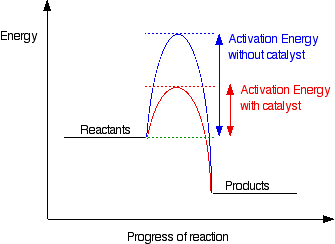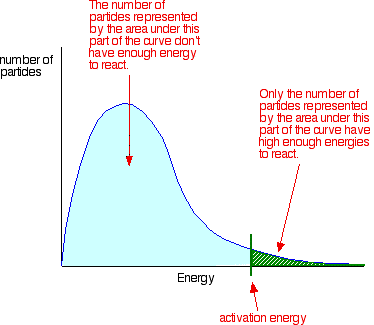# 6.1 Collision theory and rates of reaction

• Chemical Kinetics: The study and discussion of chemical reactions with respect to reaction rates
• Rate of Reaction: The change in concentration of reactants or products per unit time.

### Experimental measurements of reaction rates

• Δc, the change in concentration can be measured indeirectly by monitoring a property which changes when reactants are converted to products. Examples include:
• pH (acid – base reactions)
• Conductivity (reactions with electrolytes)
• Mass/volume (reactions involving gases)
• Colour (reactions with transition metals or other colored compounds)
• To measure reaction rate, plot concentration vs time graph. The rate is determined from slope of gradient at point t on the graph.
• Rate of reaction can be measured in three ways
• Average rate
• Avg rate = Δc/Δt
• Instantaneous rate
• Slope of tangent at a point
• Initial rate
• Slope of tangent at point t0

### Kinetic molecular theory of gases

• Explains why gases act the way they do
• Theory
1. Gases have many particles moving at high velocities in random directions
2. Size of a gaseous particle is negligible
3. Collisions between gaseous particles are elastic; no energy is lost
4. Keav is proportional to the absolute temperature in kelvin

### Occam’s razor

• Used as a guide to develop a theory
• A principle which states that “Entities should not be multiplies unnecessarily”
• This means if you have two competing theories, use the simpler one unless there is proof otherwise
• Collision theory was built using Occam’s razor

### Catalysts

• A substance that increases the rate of a chemical reaction by lowering the activation energy and is not consumed in the reaction.
• This can be demonstrated using a potential energy profile
•• Activation energy: The minimum energy required for a reaction to occur
• Catalysts come in two types:
• Homogeneous:
• In the same physical state as the reactants
• Example is destruction of ozone, O3, by chlorine atoms. In the stratosphere, ozone absorbs over 95% of UV radiation from sun. It under goes homolytic fission and converts UV radiation to heat. Chlorine atoms are produced in the reaction of a chlorofluorocarbon (CFC) with UV light.
• Heterogeneous:
• In a different state from the reactants
• Example is catalytic converter in exhaust system of car which converts harmful gaseous into water oxygen or carbon dioxide

### Maxwell – Boltzmann energy distribution and temperature

• Shows the probability of finding particles with a specific kinetic energy
• Number of particles represented by area of green (in the picture) have enough energy to react
• For a catalyzed reaction, the activation energy decrease, and there are more particles which have enough energy to react
• As temperature increases, the mean velocity of particles increases and thus the distribution become flatter and wider
•### Factors that affect the rate of chemical reaction

1. Increasing temperature
• Gives particles more kinetic energy, faster rate of reaction